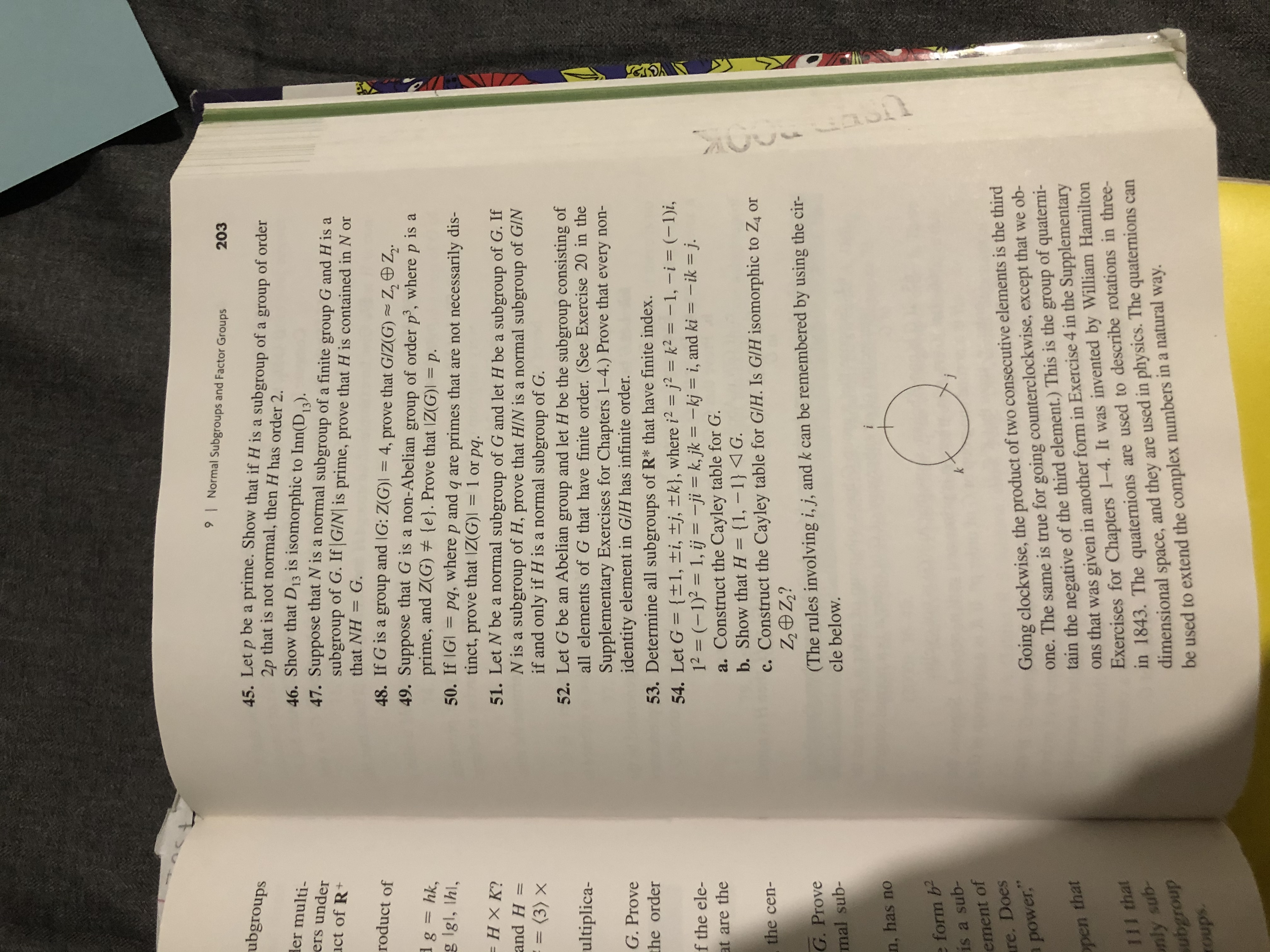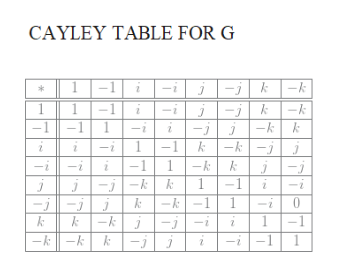# 9Normal Subgroups and Factor Groups203ubgroups45. Let p be a prime. Show that if H is a subgroup of a group of order2p that is not normal, then H has order 2.46. Show that D13 is isomorphic to Inn(D,).47. Suppose that N is a normal subgroup of a finite group G and H is asubgroup of G. If G/N| is prime, prove that H is contained in N orHer multi-ers underuct of R+that NHG.roduct of48. If G is a group and IG: Z(G) = 4, prove that G/Z(G) Z Z249. Suppose that G is a non-Abelian group of order p', where p is aprime, and Z(G) e}. Prove that IZ(G)I = p.50. If IGI= pq, where p and q are primes that are not necessarily dis-tinct, prove that IZ(G)I =51. Let N be a normal subgroup of G and let H be a subgroup of G. IfN is a subgroup of H, prove that H/N is a normal subgroup of GINif and only if H is a normal subgroup of G.52. Let G be an Abelian group and let H be the subgroup consisting ofall elements of G that have finite order. (See Exercise 20 in theSupplementary Exercises for Chapters 1-4.) Prove that every non-identity element in G/H has infinite order.53. Determine all subgroups of R* that have finite index.dg = hk,g Igl, Ihl,1 or pq.1— НХК?and H= (3) x1ultiplica-G. Provethe order54. Let G {+1, ti, tj, tk}, where i2 - j2 = k2 - -1, -i = (-1)i,12 (-1)2 1, ij = -ji= k, jk = -kj = i, and ki = -ik = j.a. Construct the Cayley table for G.f the ele-at are the(1, -1 1Gb. Show that H1c. Construct the Cayley table for G/H. Is GlH isomorphic to Z4 orZZOZ?(The rules involving i, j, and k can be remembered by using the cir-the cen-G. Provemal sub-cle below.n, has noe form bis a sub-ement ofre. Doespower,"Going clockwise, the product of two consecutive elements is the thirdone. The same is true for going counterclockwise, except that we ob-tain the negative of the third element.) This is the group of quaterni-ons that was given in another form in Exercise 4 in the SupplementaryExercises for Chapters 1-4. It was invented by William Hamiltonin 1843. The quaternions are used to describe rotations in three-dimensionalpen thatIIl thatnly sub-subgrouproups.space, and they are used in physics. The quaternions canbe used to extend the complex numbers in a natural way.uaon

Question
18 views

54help_outlineImage Transcriptionclose9 Normal Subgroups and Factor Groups 203 ubgroups 45. Let p be a prime. Show that if H is a subgroup of a group of order 2p that is not normal, then H has order 2. 46. Show that D13 is isomorphic to Inn(D,). 47. Suppose that N is a normal subgroup of a finite group G and H is a subgroup of G. If G/N| is prime, prove that H is contained in N or Her multi- ers under uct of R+ that NH G. roduct of 48. If G is a group and IG: Z(G) = 4, prove that G/Z(G) Z Z2 49. Suppose that G is a non-Abelian group of order p', where p is a prime, and Z(G) e}. Prove that IZ(G)I = p. 50. If IGI= pq, where p and q are primes that are not necessarily dis- tinct, prove that IZ(G)I = 51. Let N be a normal subgroup of G and let H be a subgroup of G. If N is a subgroup of H, prove that H/N is a normal subgroup of GIN if and only if H is a normal subgroup of G. 52. Let G be an Abelian group and let H be the subgroup consisting of all elements of G that have finite order. (See Exercise 20 in the Supplementary Exercises for Chapters 1-4.) Prove that every non- identity element in G/H has infinite order. 53. Determine all subgroups of R* that have finite index. dg = hk, g Igl, Ihl, 1 or pq. 1 — НХК? and H = (3) x 1 ultiplica- G. Prove the order 54. Let G {+1, ti, tj, tk}, where i2 - j2 = k2 - -1, -i = (-1)i, 12 (-1)2 1, ij = -ji= k, jk = -kj = i, and ki = -ik = j. a. Construct the Cayley table for G. f the ele- at are the (1, -1 1G b. Show that H 1 c. Construct the Cayley table for G/H. Is GlH isomorphic to Z4 or ZZOZ? (The rules involving i, j, and k can be remembered by using the cir- the cen- G. Prove mal sub- cle below. n, has no e form b is a sub- ement of re. Does power," Going clockwise, the product of two consecutive elements is the third one. The same is true for going counterclockwise, except that we ob- tain the negative of the third element.) This is the group of quaterni- ons that was given in another form in Exercise 4 in the Supplementary Exercises for Chapters 1-4. It was invented by William Hamilton in 1843. The quaternions are used to describe rotations in three- dimensional pen that IIl that nly sub- subgroup roups. space, and they are used in physics. The quaternions can be used to extend the complex numbers in a natural way. uaon fullscreen
check_circle

Step 1

The problem concerns the groujp properties of the Quaternion group of order 8,

Step 2

a)Cayley table for G. Let G be as given. For the product xy, x is read from the column and y read from the row. For example , ij = k (i from 4th row and j from 6th column), Note that order is important , as the group is non-commutative. ij =k , whereas ji =-khelp_outlineImage TranscriptioncloseCAYLEY TABLE FOR G kk -1 -1 -k 1 -k -k 1 -1 j -1 1 -i -1k 1 -k-1 -1 kk j -1 -k -i -k -k fullscreen
Step 3

b)  From the table, -1 and (of course , the identity element ...

### Want to see the full answer?

See Solution

#### Want to see this answer and more?

Solutions are written by subject experts who are available 24/7. Questions are typically answered within 1 hour.*

See Solution
*Response times may vary by subject and question.
Tagged in

### Math